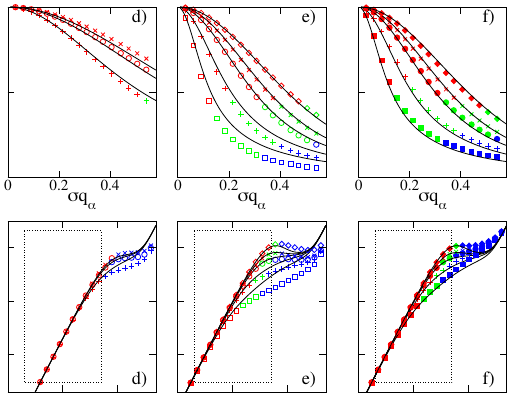Scattering from networks

Paper: "Scattering from polymer networks under elongational strain" Europhysics Letters 72, 760 (2005). Authors: C. Svaneborg,  G. S. Grest and R. Everaers

Molecular-dynamics simulations are used to sample the single-chain form factor of labelled sub-chains in model polymer networks under elongational strain. We observe very similar results for randomly cross-linked and for randomly end-linked networks with the same average strand length and see no indication of lozenge-like scattering patterns reported for some experimental systems. Our data analysis shows that a recent variant of the tube model quantitatively describes scattering in the Guinier regime as well as the macroscopic elastic properties. The observed failure of the theory outside the Guinier regime is shown to be due to non-Gaussian pair-distance distributions.The figure above shows predicted small-angle Neutron scattering spectra for deformed end-linked networks randomly isotope labelled with labels matching the strand length. The top row is the normalized form factor, while the bottom row shows a Kratky representation. The box illustrates the experimentally available q range. The left and middle plots correspond to networks with strand length=label length 20 (left) and 100 (middle). The right plot is the predicted scattering assuming Gaussian statistics applies at all length scales, i.e. neglecting the fact that monomers have finite size are packed locally. The solid lines are predictions for the scattering using the Double Tube model. Finally the color of the symbols denote the length scale larger than the radius of gyration (red), inside the tube (blue), and intermediate length scales (green).

We observe perfect agreement between the simulation results and theory in the right column. This is an artifact due to the fact that the theory is based on Gaussian chain statistics at all length scales, and we doctored the simulation data to display the same artifact. Hence the deviations between theory and simulation data are due to monomeric packing also present in real systems.

Hence we conclude that when fitting real scattering data (left and middle column) with a tube model (solid line, all tube models are based on the assumption of Gaussian chain statics) one should be careful to pick only data on large length scales (red) and for small deformations for intermediate deformations (green), where the theory can reliably describe the simulation data. Naively fitting a tube model to scattering data on all length scales will create systematic errors due to the failure of the theory in describing data at intermediate length scales for large deformations and data measured at length scales inside the tube.